•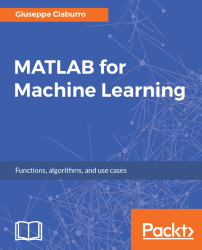#### MATLAB for Machine Learning#### Overview of this book

MATLAB is the language of choice for many researchers and mathematics experts for machine learning. This book will help you build a foundation in machine learning using MATLAB for beginners. You’ll start by getting your system ready with t he MATLAB environment for machine learning and you’ll see how to easily interact with the Matlab workspace. We’ll then move on to data cleansing, mining and analyzing various data types in machine learning and you’ll see how to display data values on a plot. Next, you’ll get to know about the different types of regression techniques and how to apply them to your data using the MATLAB functions. You’ll understand the basic concepts of neural networks and perform data fitting, pattern recognition, and clustering analysis. Finally, you’ll explore feature selection and extraction techniques for dimensionality reduction for performance improvement. At the end of the book, you will learn to put it all together into real-world cases covering major machine learning algorithms and be comfortable in performing machine learning with MATLAB.
Title PageCreditsForewordwww.PacktPub.comCustomer FeedbackPrefaceFree Chapter
Getting Started with MATLAB Machine LearningImporting and Organizing Data in MATLABFrom Data to Knowledge DiscoveryFinding Relationships between Variables - Regression TechniquesPattern Recognition through Classification AlgorithmsIdentifying Groups of Data Using Clustering MethodsSimulation of Human Thinking - Artificial Neural NetworksImproving the Performance of the Machine Learning Model - Dimensionality ReductionMachine Learning in Practice## Discover the different types of machine learning

The power of the machine learning is due to the quality of its algorithms, which have been improved and updated over the years; these are divided into several main types depending on the nature of the signal used for learning or the type of feedback adopted by the system. They are:

• Supervised learning: The algorithm generates a function that links input values ​​to a desired output through the observation of a set of examples in which each data input has its relative output data, and that is used to construct predictive models.
• Unsupervised learning : The algorithm tries to derive knowledge from a general input without the help of a set of pre-classified examples that are used to build descriptive models. A typical example of the application of these algorithms are search engines.
• Reinforcementlearning:  The algorithm is able to learn depending on the changes that occur in the environment in which it is performed. In fact, since every action has some effect on the environment concerned, the algorithm is driven by the same feedback environment. Some of these algorithms are used in speech or text recognition.

The following is a figure depicting the different types of machine learning algorithms: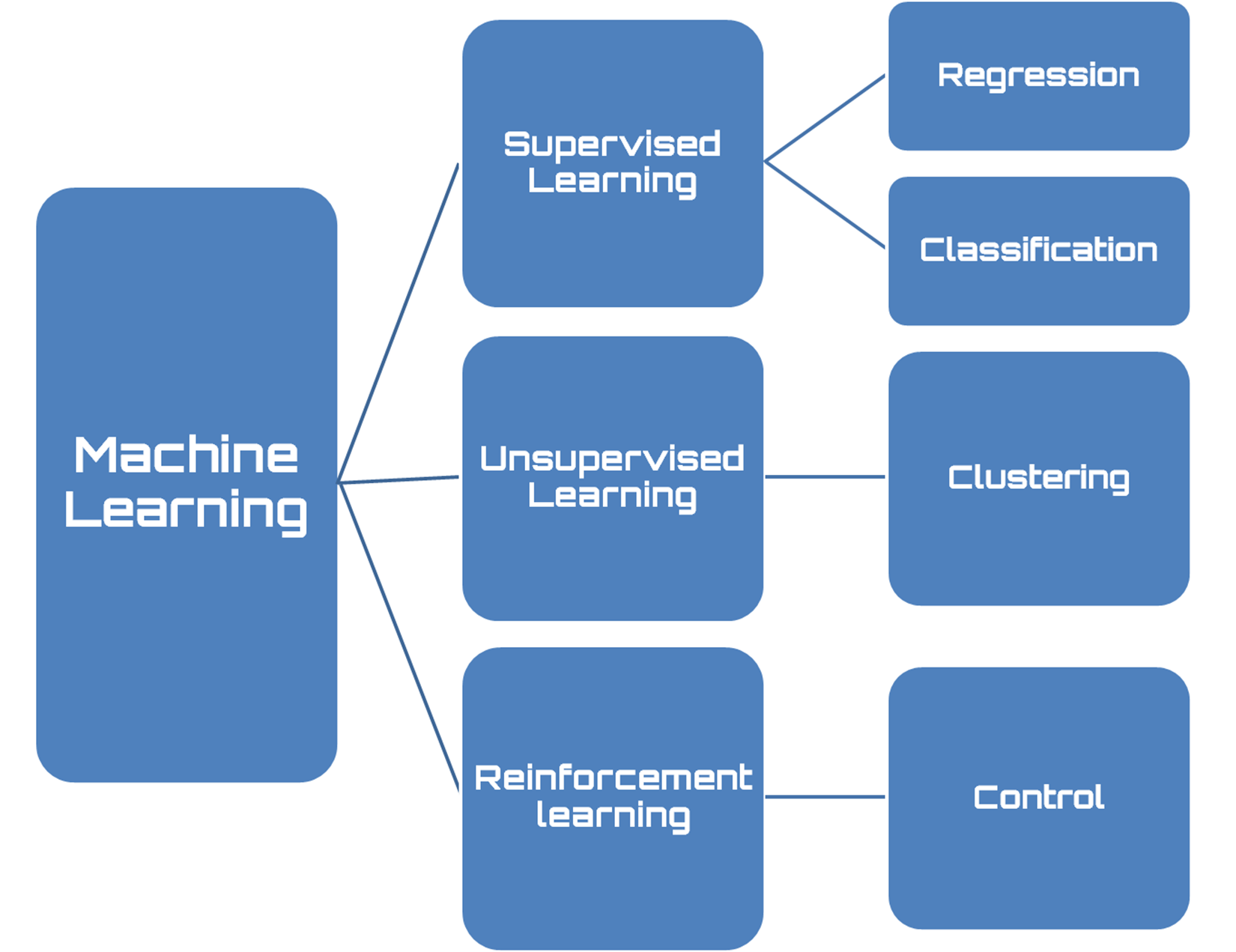Figure 1.5: Types of machine learning algorithms

### Supervised learning

Supervised learning is a machine learning technique that aims to program a computer system so that it can resolve the relevant tasks automatically. To do this, the input data is included in a set I, (typically vectors). Then the set of output data is fixed as set O, and finally it defines a function f that associates each input with the correct answer. Such information is called a training set. This workflow is presented in the following figure: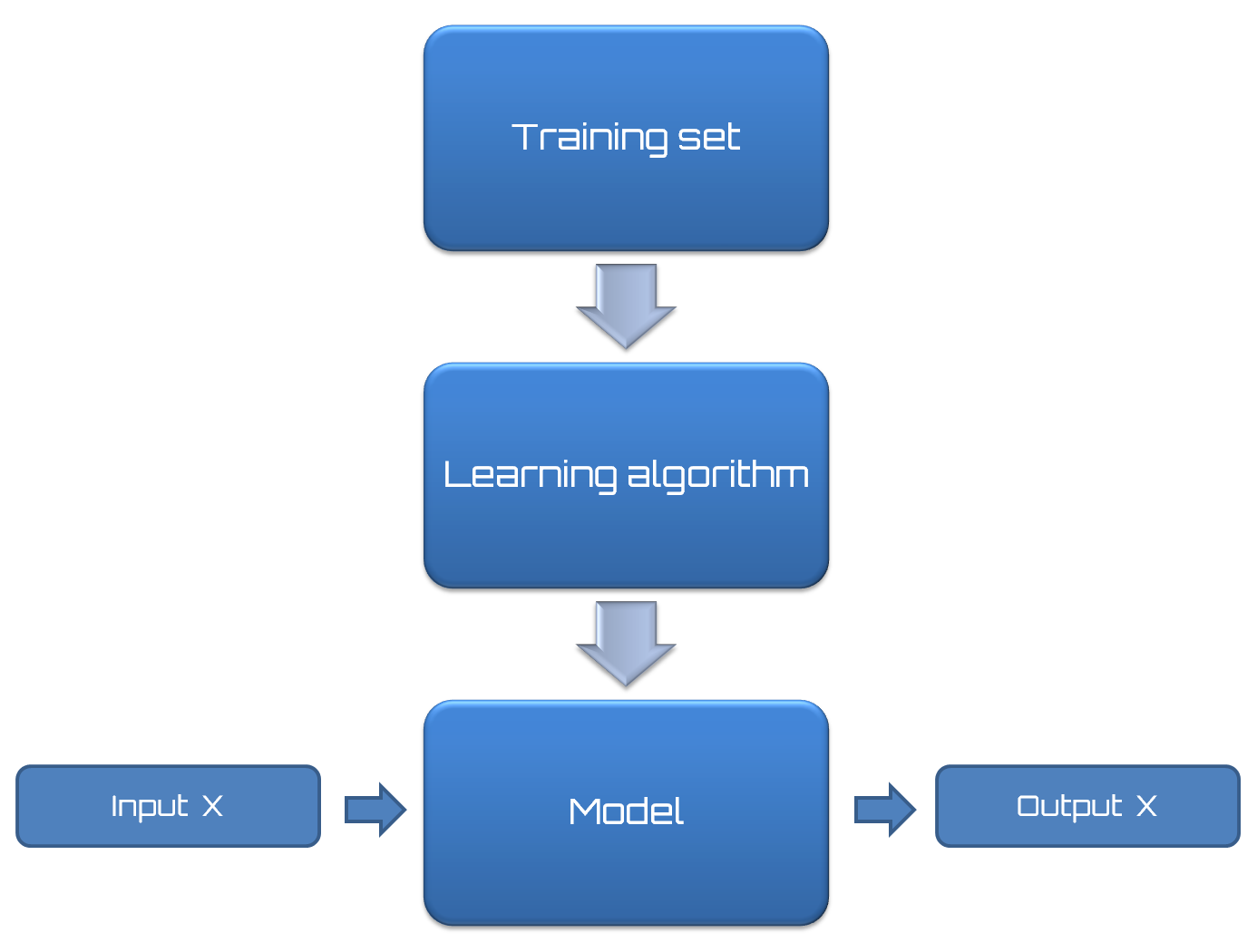Figure 1.6: Supervised learning workflow

All supervised learning algorithms are based on the following thesis: if an algorithm provides an adequate number of examples, it will be able to create a derived function B that will approximate the desired function A.

If the approximation of the desired function is adequate, when the input data is offered to the derived function, this function should be able to provide output responses similar to those provided by the desired function and then acceptable.These algorithms are based on the following concept: similar inputs correspond to similar outputs.Generally, in the real-world, this assumption is not valid; however, some situations exist in which it is acceptable. Clearly, the proper functioning of such algorithms depends significantly on the input data. If there are only a few training inputs, the algorithm might not have enough experience to provide a correct output. Conversely, many inputs may make it excessively slow since the derivative function generated by a large number of inputs could be very complicated.

Moreover, experience shows that this type of algorithm is very sensitive to noise; even a few pieces of incorrect data can make the entire system unreliable and lead to wrong decisions.

In supervised learning, it's possible to split problems based on the nature of the data. If the output value is categorical, such as membership/non-membership to a certain class, it is a classification problem. If the output is a continuous real value in a certain range, then it is a regression problem.

### Unsupervised learning

The aim of unsupervised learning is to automatically extract information from databases. This process occurs without prior knowledge of the contents to be analyzed. Unlike supervised learning, there is no information on membership classes of the examples or generally on the output corresponding to a certain input. The goal is to get a model that is able to discover interesting properties: groups with similar characteristics (clustering) for instance. Search engines are an example of an application of these algorithms. Given one or more keywords, they are able to create a list of links related to our search.

The validity of these algorithms depends on the usefulness of the information they can extract from the databases. These algorithms work by comparing data and looking for similarities or differences. Available data concerns only the set of features that describe each example.

The following figure shows supervised learning and unsupervised learning examples: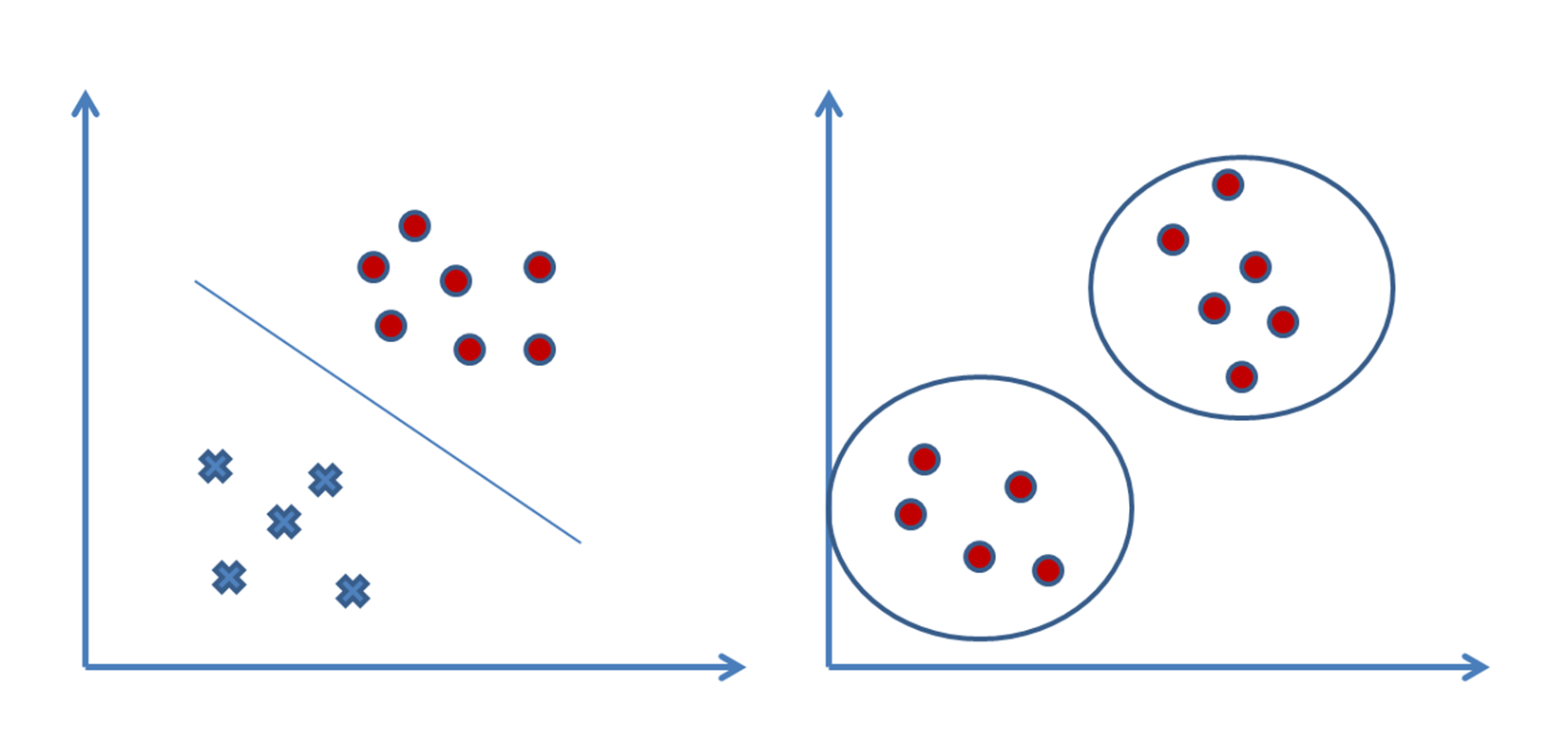Figure 1.7: Supervised learning versus unsupervised learning

They show great efficiency with elements of numeric type, but are much less accurate with non-numeric data. Generally, they work properly in the presence of data that contains an order or a clear grouping and is clearly identifiable.

### Reinforcement learning

Reinforcement learning aims to create algorithms that can learn and adapt to environmental changes. This programming technique is based on the concept of receiving external stimuli depending on the algorithm choices. A correct choice will involve a premium while an incorrect choice will lead to a penalty. The goal of system is to achieve the best possible result, of course.

In supervised learning, there is a teacher that tells the system which is the correct output (learning with a teacher). This is not always possible. Often we have only qualitative information (sometimes binary, right/wrong, or success/failure).

The information available is called reinforcement signals. But the system does not give any information on how to update the agent's behavior (that is, weights). You cannot define a cost function or a gradient. The goal of the system is to create the smart agents that have a machinery able to learn from their experience.

This flowchart shows reinforcement learning: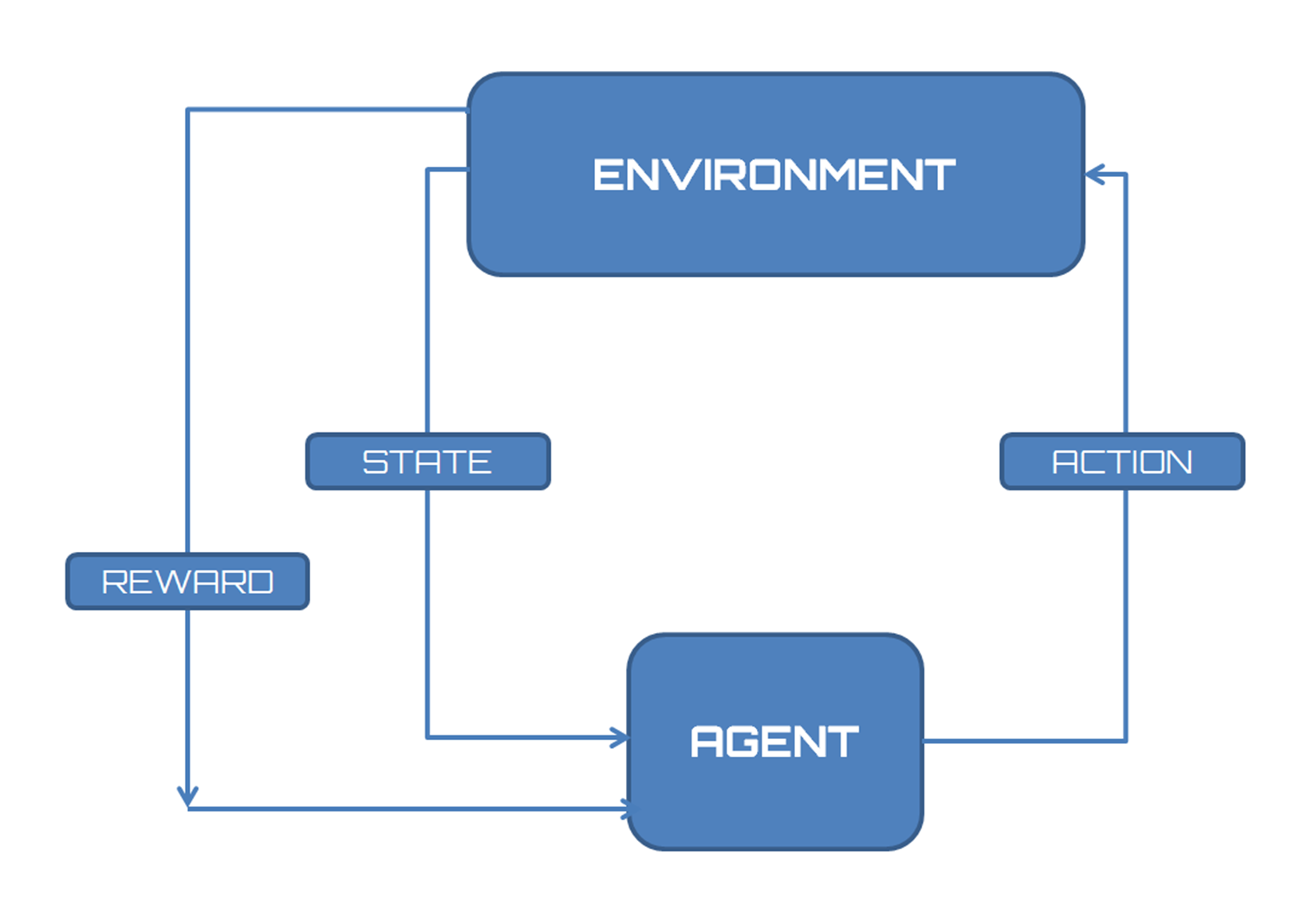Figure 1.8: How to reinforcement learning interact with the environment